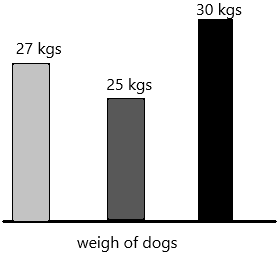Practice the problems of Math in Focus Grade 2 Workbook Answer Key Chapter 8 Mass to score better marks in the exam.

Math Journal

Look at the pictures.

Question 1.
Write sentences to compare the mass of the boxes. Use the words lighter, heavier, lightest and heaviest.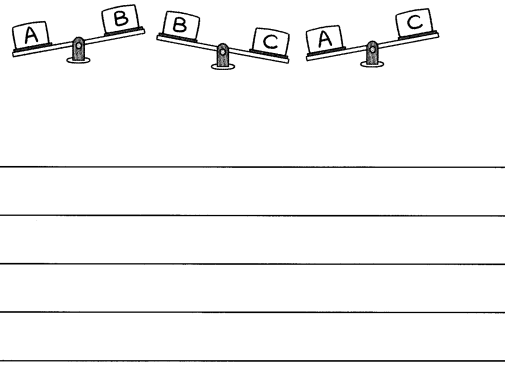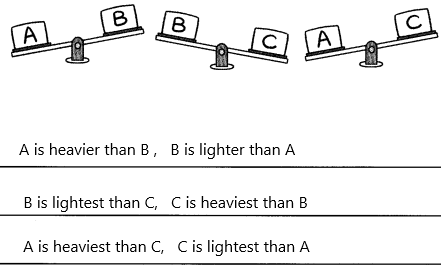1. The weight of an object is a force that pushes downwards. You can feel the weight of lots of different objects when you hold them. It is the reason we find it difficult to lift some objects at all.
2. Below is a set of scales. Scales can be used to compare the weights of two objects.
3. We place a mass at one end of the scale. There is nothing on the other end.
4. The weight of mass pushes down on this end of the scale and so this left side moves downwards.
5. This causes the other side to rise upwards.

Question 2.
Order the boxes from heaviest to lightest.
___, ____, ____
Explanation:
The heaviest object moves to scale downward.
The lightest object moves upward.

Write True or False.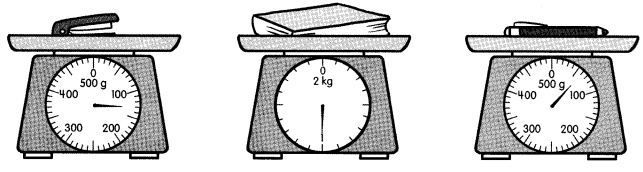Example
The stapler is as heavy as the pen.
False

Question 3.
The pen has a mass of 60 grams. _____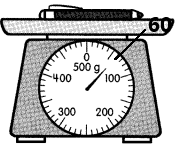Question 4.
The stapler is 70 grams lighter than the pen. ____
Explanation:
The mass of a stapler is 130 grams.
The mass of the pen is 60 grams.
The mass of the stapler is heavier than the mass of the pen.

Question 5.
The book is the heaviest. ___
The mass of the book is about 1 kg.

Question 6.
The total mass of the stapler and the pen is 190 grams. ____
Explanation:
The mass of a stapler is 130 grams.
The mass of the pen is 60 grams.
The total mass=130+60=190.
Therefore, the total mass of both stapler and pen is 190 grams.

Challenging Practice

Fill in the blanks.

Question 1.
In Picture A, 3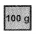are needed to make the needle on the scale point as shown. How many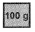are needed to make the needle point as shown in Picture B?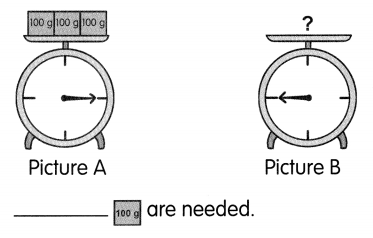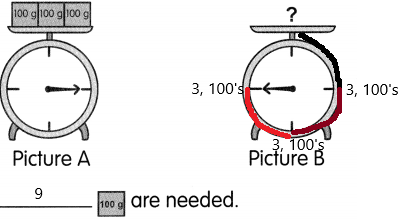For picture A, 3 1oog are needed.
For picture B, I represented the below add all the 3’s.
3+3+3=9
therefore, 9 100’s are needed.

Question 2.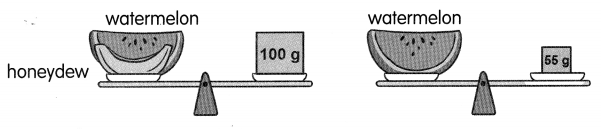The slice of honeydew has a mass of ___ grams.
Explanation:
1 slice of watermelon is 55 grams.
I honeydew and watermelon slice both are having a mass of 100 grams.
We need to calculate honeydew slices. assume it as X.
X=100-55
X=45
Therefore, the honeydew slice is 45 grams.

Question 3.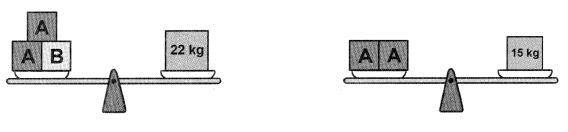Box B has a mass of ___ kilograms.
Explanation:
In 1st picture, 22 kgs are given.
The mass of two A’s is 15 kgs.
So the remaining kgs are B.
To calculate the mass of B we need to subtract total kgs and two A’s mass. Assume it as X.
X=22-15
X=7
therefore, the mass of bag B is 7 kgs.

Solve.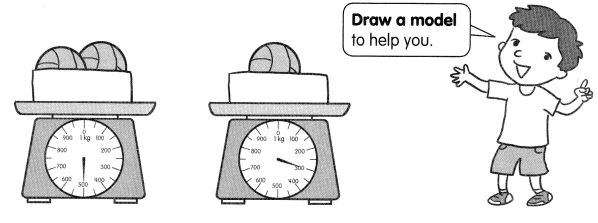a. What is the mass of one ball? ___ g
Explanation:
Already given in the first picture, the total balls and box are having 500 grams. Suppose If we take each ball 200 grams then 2 balls will be 200*2=400.
Therefore, the mass of one ball is 200 grams.

b. What is the mass of the box? ___ g
Explanation:
Already given in the first picture, the total balls and box are having 500 grams. Suppose If we take each ball 200 grams then 2 balls will be 200*2=400. Now coming to the mass of the box is 100 grams.
How 100 grams?
The mass of the two balls is 400 and the remaining 100 grams is the mass of the box.

Chapter Review/Test

Vocabulary

Fill in the blanks with the words below.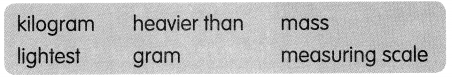Question 1.
A table is ___ a watch.
a table is heavier than a watch.

Question 2.
A ___ is a bigger unit of mass and a ___ is a smaller unit of mass.
A kilogram is a bigger unit of mass.
A Metric measure of mass (which we feel as weight).
The abbreviation is kg.
1 kg = 1000 grams.
1 kg = 2.205 pounds (approximately).
Example: The gold bar has a mass of 1 kg.
A gram is a smaller unit of mass.
A Metric measure of mass (which we feel as weight)
A paperclip weighs about 1 gram
Abbreviation is g
1000 grams = 1 kilogram

Question 3.
To measure how heavy an object is, you find its ____
Mass is a measure of the amount of matter inside an object. An object can be quite small yet has a lot of matter inside of it.
A bar of gold for instance may be quite small and yet has a mass of 2 kilograms (kg)
Talking about kilograms, what is 1 kilogram?
One kilogram is the mass of 1 litre of water in its densest state.
Water is in its densest state when the temperature is close to 4 degrees Celsius.
The following cube will be able to hold 1 litre of water.The volume of the cube = 10 × 10 × 10 = 1000 cm3
In math 1 liter = 1000 cm3

Question 4.
You use a ___ to measure the mass of an object.
Explanation:
Scales of measurement in math are used to classify and/or quantify variables based on certain properties. Each grade of measurement has relevant properties that are crucial to know. The scale of measurement in math is commonly interpreted in the form of graphs. This can be described as the mechanism of marks at fixed intervals, which clearly explain the link between the units being used and their illustration on the graph. Data of Measurement scales are basically classified under the four scales of measurement that have frequent applications in statistical analysis:
The 4 types of scales of measurement include:-
1. Nominal Scale of Measurement
2. Ordinal Scale of Measurement
3. Interval Scale of Measurement
4. Ratio scales Scale of Measurement.

Question 5.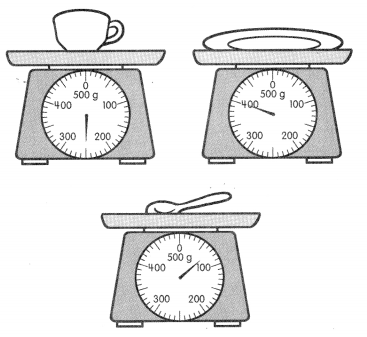The spoon is the ___
Explanation:
The cup is having a mass of 250 grams.
The plate is a mass of 400 grams.
The spoon is a mass of 70 grams.
So the spoon is the lightest.

Concepts and Skills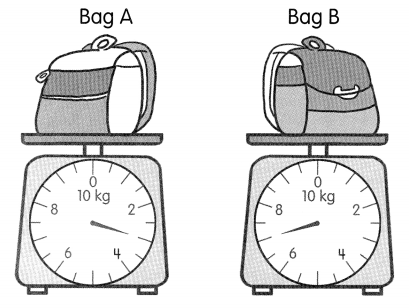Question 6.
Which bag is heavier? Bag ____
Explanation:
Observe the picture carefully, and then write the masses of their respective bags.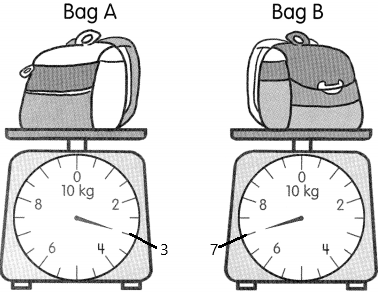The mass of bag A is 3 kgs
The mass of bag B is 7 kgs.
Here asked the bag which one is heavier.
7>3.
Bag B is having 7kgs which is heavier.

Question 7.
How much heavier is it? ___ kgThe bag B is 7 kgs heavier.

Question 8.
What is the total mass of both bags? ___ kg
The mass of bag A is 3 kgs
The mass of bag B is 7 kgs
The total of both the bags=X
X=3+7
X=10
therefore, both bags combine having 10 kgs.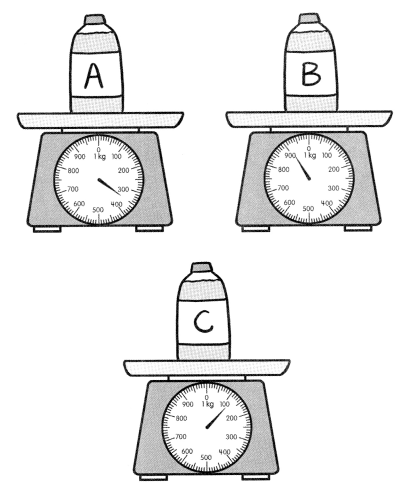Question 9.
Which bottle is the lightest? Bottle ____
Explanation:
The mass of bottle A is 350 grams.
The mass of bottle B is 900 grams.
The mass of bottle C is 120 grams.
By comparing all the bottles the lightest one is 120 grams.
So, bottle C is the lightest.

Question 10.
What is the difference in mass between the heaviest and lightest bottle? ___ g
Explanation: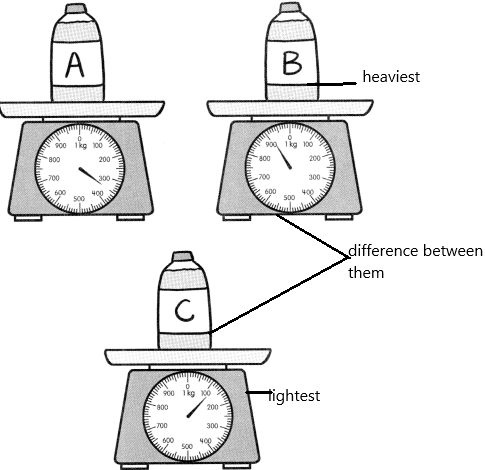The mass of bottle B is 900 grams which are the heaviest.
The mass of bottle C is 120 grams which are lighter.
To find the difference between both the masses we need to subtract them. Assume it as X. Then we get the answer.
X=900-120
X=780
Therefore, the difference is 780 grams.

Question 11.
What is the total mass of Bottle A and Bottle C? ____ g
Explanation: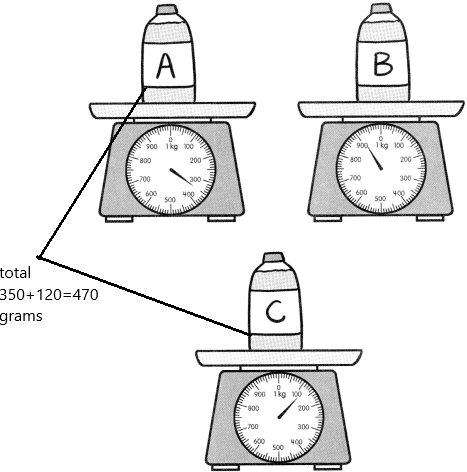The mass of bottle A is 350 grams.
The mass of bottle C is 120 grams.
To find the total we need to add both the masses. Assume it as X.
X=350-120
X=470
Therefore, the total mass is 470 grams.

Question 12.
Order the bottles from lightest to heaviest.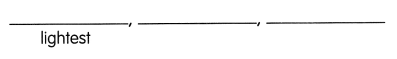Explanation: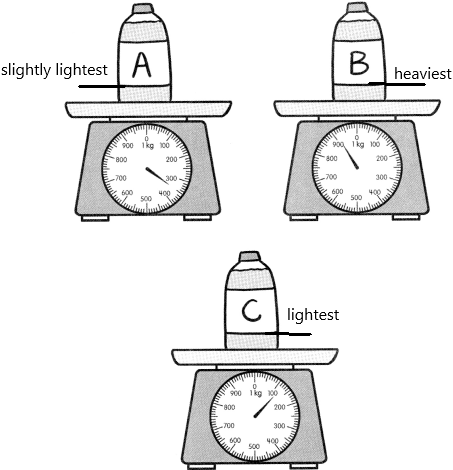It was like ascending order. It means we need to order from lowest to highest.

Problem Solving

Solve.

Question 13.
Mr. Shepherd has 5 kilograms of rice. He buys another 8 kilograms of rice. How many kilograms of rice does he have?
Mr. Shepherd has ____ kilograms of rice.
Explanation:
The number of kilograms of rice Shepherd has=5
The number of kilograms of rice he buys extra=8
The total number of kilograms he has now=X
To get the total kilograms of rice we need to add both 5 and 8.
X=8+5
X=13
Therefore, 13 kgs of rice he has now.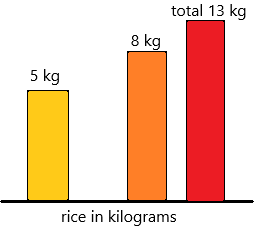Question 14.
Claudia has two boxes. The mass of Box A is 980 grams. The mass of Box B is 750 grams. What is the difference in masses between the two boxes?
The difference in masses between the two boxes is ___ grams.
Explanation:
The mass of box A=980 grams.
The mass of box B=750 grams.
The difference between two boxes=X
Subtract both the masses to get the answer.
X=980-750
X=230
Therefore, the difference between the two boxes is 230 grams.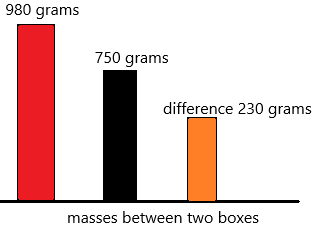Question 15.
Casey has 500 grams of carrots. He buys another 400 grams of carrots. He uses 725 grams of carrots for a recipe. How many grams of carrots does he have left?
Casey has ___ grams of carrots left.
Explanation:
The number of grams of carrots Casey has=500
The number of grams of carrots he buys extra=400
The total number of grams he has now=500+400=900
The number of grams of carrots he used for recipe=725.
We need to calculate the number of grams of carrots he has left with him after using for the recipe. Assume it X.
Subtract the total number of grams of carrots and use carrots.
X=900-725
X=175
Therefore, Casey has 175 grams of carrots.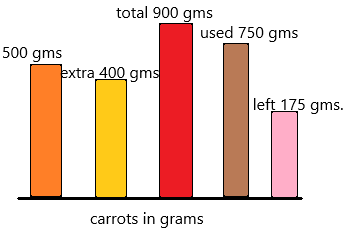Question 16.
Lily’s dog weighs 27 kilograms. Her dog is 2 kilograms heavier than Ben’s dog. Joe’s dog is 5 kilograms heavier than Ben’s dog. What is the mass of Joe’s dog?
The mass of Joe’s dog is ___ kilograms.